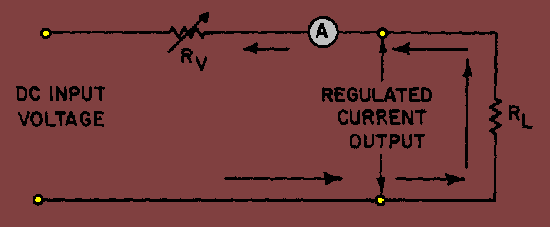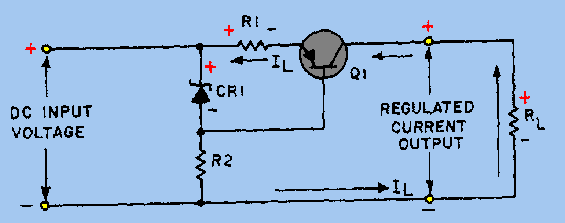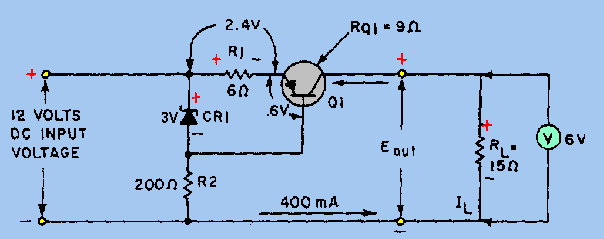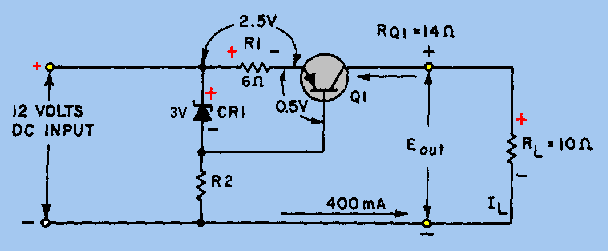circuitry which provides a constant current output is called a constant current regulator or just CURRENT REGULATOR. The schematic shown in figure 4-40 is a simplified schematic for a current regulator. The variable resistor shown on the schematic is used to illustrate the concept of current regulation.">Custom SearchCurrent Regulators You should now know how voltage regulators work to provide constant output voltages. In some circuits it may be necessary to regulate the current output. The circuitry which provides a constant current output is called a constant current regulator or just CURRENT REGULATOR. The schematic shown in figure 4-40 is a simplified schematic for a current regulator. The variable resistor shown on the schematic is used to illustrate the concept of current regulation. You should know from your study of voltage regulators that a variable resistor does not respond quickly enough to compensate for the changes. Notice that an ammeter has been included in this circuit to indicate that the circuit shown is that of a current regulator. When the circuit functions properly, the current reading of the ammeter remains constant. In this case the variable resistor (RV) compensates for changes in the load or dc input voltage. Adequate current regulation results in the loss of voltage regulation. Studying the schematic shown, you should recall that any increase in load resistance causes a drop in current. To maintain a constant current flow, the resistance of RV must be reduced whenever the load resistance increases. This causes the total resistance to remain constant. An increase in the input voltage must be compensated for by an increase in the resistance of RV, thereby maintaining a constant current flow. The operation of a current regulator is similar to that of a voltage regulator. The basic difference is that one regulates current and the other regulates voltage. Figure 4-40. - Current regulator (simplified).Since use of a variable resistor is not a practical way to control current fluctuation or variation, a transistor and a Zener diode, together with necessary resistors, are used. Recall that the Zener diode provides a constant reference voltage. The schematic shown in figure 4-41 is that of a current regulator circuit. Except for the addition of R1, the circuit shown in the figure is similar to that of a series voltage regulator. The resistor is connected in series with the load and senses any current changes in the load. Notice the voltage drop across R1 and the negative voltage polarity applied to the emitter of Q1. The voltage polarity is a result of current flowing through R1, and this negative voltage opposes the forward bias for Q1. However, since the regulated voltage across CR1 has an opposite polarity, the actual bias of the transistor is the difference between the two voltages. You should see that the purpose of R2 is to function as a current-limiting resistor for the Zener diode. Figure 4-41. - Current regulator.The purpose of a current regulator is to provide a constant current regardless of changes in the input voltage or load current. The schematic shown in figure 4-42 is that of a circuit designed to provide a constant current of 400 milliamperes. Voltmeters are shown in the schematic to emphasize the voltage drops across specific components. These voltages will help you understand how the current regulator operates. The voltage drop across the base-emitter junction of Q1 is 0.6 volt. This voltage is the difference between the Zener voltage and the voltage drop across R1. The 0.6-volt forward bias of Q1 permits proper operation of the transistor. The output voltage across RL is 6 volts as shown by the voltmeter. With a regulated current output of 400 milliamperes, the transistor resistance (RQ1) is 9 ohms. This can be proved by using Ohm's law and the values shown on the schematic. In this case, current (I) is equal to the voltage drop (E) divided by the resistance (R). Therefore: 12 volts divided by 30 ohms equals 0.4 ampere, or 400 milliamperes. Figure 4-42. - Current regulator (with circuit values).Since you are familiar with the basic current regulating circuitry, let's examine in detail how the various components work to maintain the constant 400-milliampere output. Refer to the schematic shown in figure 4-43. Remember a decrease in load resistance causes a corresponding increase in current flow. In the example shown, the load resistance RL has dropped from 15 ohms to 10 ohms. This results in a larger voltage drop across R1 because of the increased current flow. The voltage drop has increased from 2.4 volts to 2.5 volts. Of course, the voltage drop across CR1 remains constant at 9 volts due to its regulating ability. Because of the increased voltage drop across R1, the forward bias on Q1 is now 0.5 volt. Since the forward bias of Q1 has decreased, the resistance of the transistor increases from 9 ohms to 14 ohms. Notice that the 5 ohm increase in resistance across the transistor corresponds to the 5 ohm decrease in the load resistance. Thus, the total resistance around the outside loop of the circuit remains constant. Since the circuit is a current regulator, you know that output voltages will vary as the regulator maintains a constant current output. In the figure, the voltage output is reduced to 4 volts, which is computed by multiplying current (I) times resistance (R) (400 mA X 10 ohms = 4 volts). Figure 4-43. - Current regulator (with a decrease in RL).Q.36 In figure 4-40, when there is an increase in the load resistance (R L), the resistance of R V increases/decreases(which one) to compensate for the change.Q.37 In figure 4-43 any decrease in the base-emitter forward bias across Q1 results in an increase/a decrease (which one) in the resistance of the transistor.Integrated Publishing, Inc. - A (SDVOSB) Service Disabled Veteran Owned Small Business